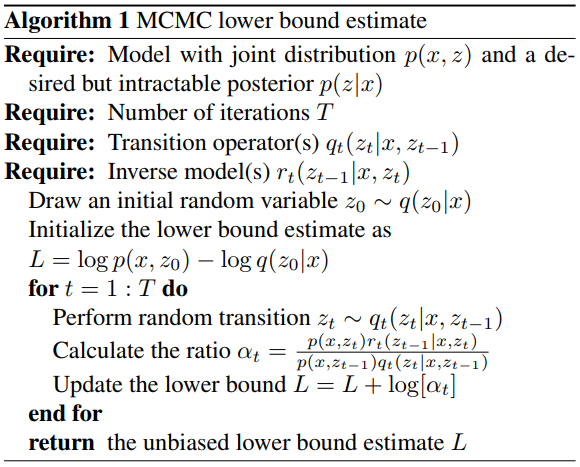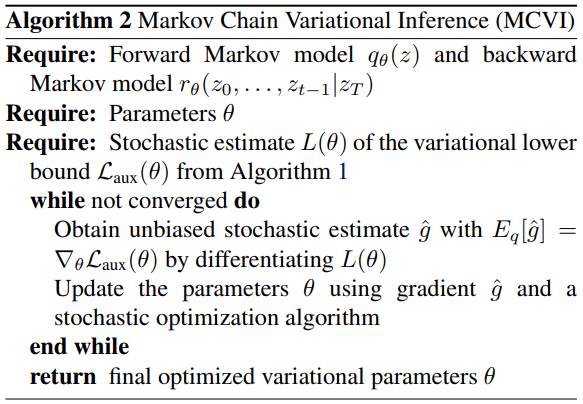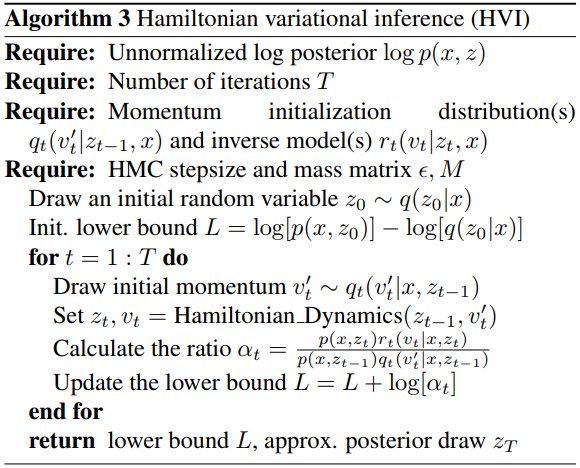# Markov Chain Monte Carlo and Variational Inference: Bridging the Gap

## WHY?

Two approximation methods, Variational inference and MCMC, have different advantages: usually, variational inference is fast while MCMC is more accurate.

## Note

Markov Chain Monte Carlo (MCMC) is approximation method of estimating a variable. MCMC first sample a random draw z_0$z_0$ and than draw a chain of variables from a stochastic transition operator q.

z_t \sim q(z_t|z_{t-1},x)
 It is proved that z_T$z_T$ will ultimately converge to true posterior p(z x) with sufficiently enough samplings.

## WHAT?

Variational lowerbound contains posterior of latent variable q(z|x). This paper suggests to estimate q(z|x) more accurately with MCMC with auxiliary random variables and apply variational inference to ELBO with auxiliary variables. \

y = z_0, z_1, ..., z_{t-1}\\
L_{aux} = \mathbb{E}_{q(y,z_T|x)}[\log[p(x,z_T)r(y|x,z_T)] - \log q(y, z_T|x)]\\
\mathcal{L} - \mathbb{E}_{q(z_T|x)}\{D_{KL}[q(y|z_T,x)\|r(y|z_T,x)]\}\leq \mathcal{L} \leq \log[p(x)]\\

If we assume that auxiliary inference distribution also has a Markov structure,

r(z_0, ... z_{t-1}|x, z_T) = \prod^T_{t=1}r_t(z_{t-1}|x, z_t)\\
\log p(x) \leq \mathbb{E}_z[\log p(x, z_T) - \log q(z_0, ..., z_T|x) + \log r(z_0, ... z_{t-1}|x, z_T)]\\
= \mathbb{E}_q[log[p(x, z_T)/q(z_0|x)] + \sum_{t=1}^T\log[r_t(z_{t-1}|x, z_t)/q_t(z_t|x,z_{t-1})]]

Since auxiliary variational lowerbound cannot be calculated analytically, we estimate MCMC lowerbound by sampling from transitions(q_t$q_t$) and inverse model(r_t$r_t$).The gradient of resulted MCMC lowerbound can be calculated via remarameterization trick.One of the efficient methods of MCMC is Hamiltonian Monte Carlo (HMC). Hamiltonian MC introduce auxiliary variables v with the same dimension as z.

H(v, z) = 0.5 v^T M^{-1}v - \log p(x,z)\\
v_t' \sim q(v_t'|x, z_{t-1})\\## So?

Convolutional VAE with Hamiltonian Variational Inference (HVI) with 16 leapfrog step and 800 hidden nodes achieve slightly worse result than that of DRAW.

## Critic

Many MCMC algorithms variates can be used to improve the result.

Powered by aiden